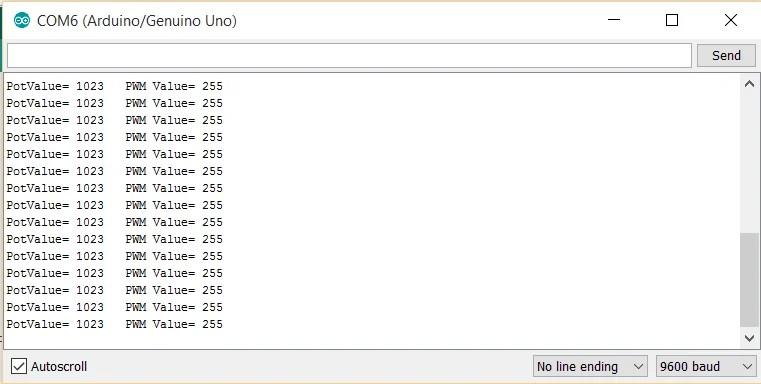# Arduino Programming for absolute Beginners in 2018: The Ultimate Guide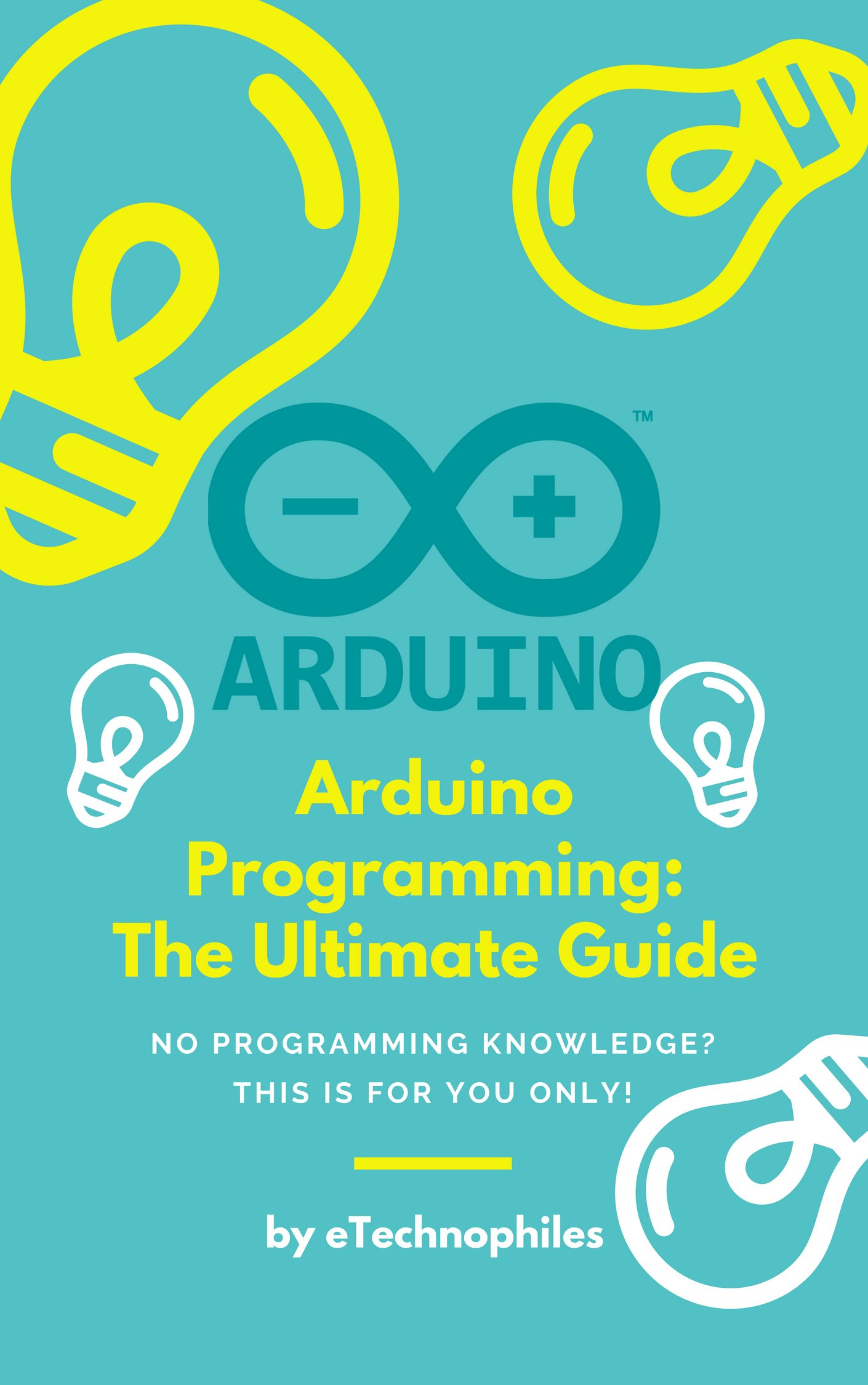If you are new to Arduino and have no prior knowledge of programming, then this “ Arduino Programming for absolute Beginners in 2018: The Ultimate Guide ” is for you only. In this post you will learn everything you need to know about Arduino programming in order to build those mind-boggling projects. This detailed guide is filled with examples, so you can grab the concepts more clearly.

What is Arduino?

Arduino is an Italy based company that manufactures Microcontroller boards called Arduino Boards which are used in many electronics and day to day applications around the world.

Arduino is one of the most popular Microcontroller based Development Board on Earth since many Electronics hobbyists and students from all over the world use Arduino Boards in their day to day projects. One of the key reasons for Arduino being so popular is that it is Open Source Software and Open Source Hardware-based Board.

One of the factors that separate Arduino from all the other Microcontroller boards out there is its community of thousands of users. Queries, Projects, and questions are posted daily on the Internet by its community members. Checkout: Arduino forum

Why go for Arduino?

A) Open Source Software: You can alter the software or code according to your need or can design an entirely new environment for Arduino without any software copyright issue.

B) Open Source Hardware: You can change the Hardware circuitry of Arduino boards according to your need or can design an entirely new  Hardware system on Arduino without any Arduino Hardware copyright issue.

C) Large Community of Thousands of users.

This is answered best in Quora:  Why is Arduino so popular?

Types of Arduino Boards:

Since there are so many applications and areas where Microcontroller board like Arduino Can be used, it is impossible to fit all features in one type of Arduino Board. Hence there are many types of Arduino boards available in the market according to application and user’s need.

Some of the popular Arduino Boards are listed below:India: https://amzn.to/2miGBMmIndia: https://amzn.to/2NdS9McIndia: https://amzn.to/2LfB0ER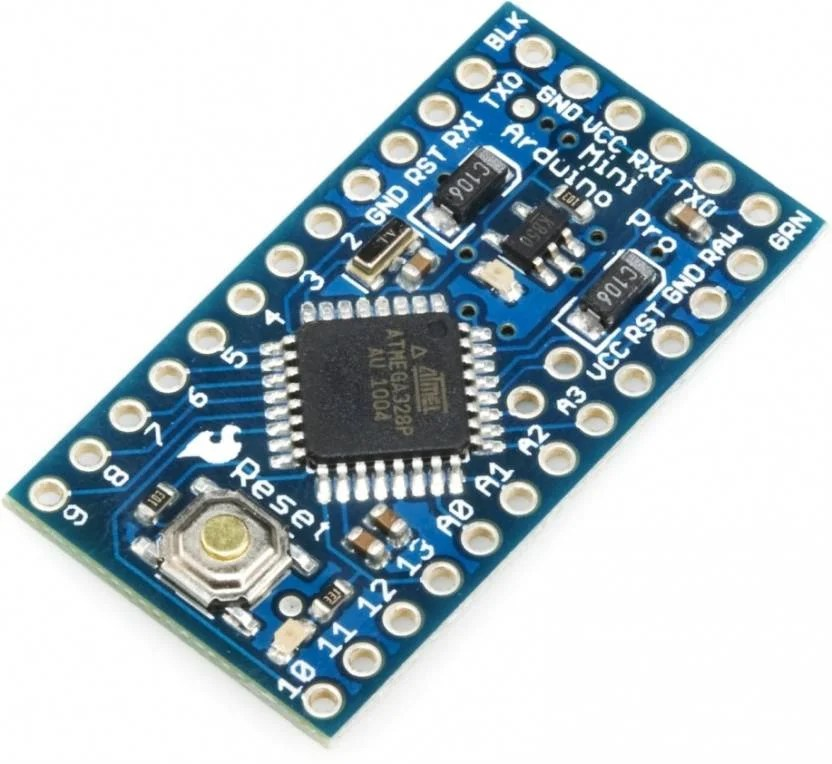India: https://amzn.to/2LjrHAe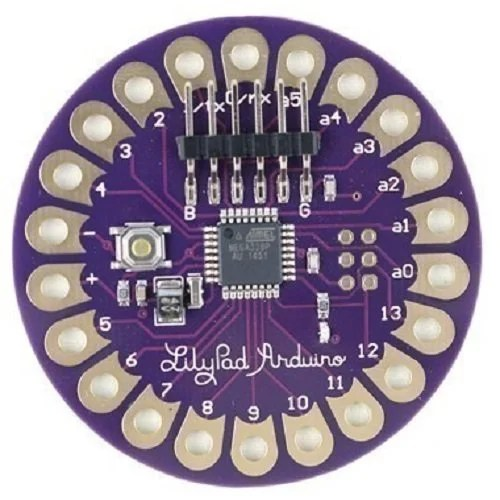India: https://amzn.to/2N9DHEU

The Simplest Arduino Board for Beginners: Arduino UNOAmong all types of Arduino Boards, Arduino UNO is the most popular one. It is a best option for Arduino beginners who are just starting out their journey in the field of Microcontrollers based Projects and Automation. Anyone can start making projects using Arduino UNO just after a few minutes of reading and practicing.

Best Arduino kits for Beginners:

There are many Arduino kits available for beginners in the Market. We have compiled the list of top Arduino kits available on Amazon for beginners in the following posts. You can checkout them later after reading this Guide.

Arduino Programming Basics:

What is Arduino IDE( Integrated Development Environment) ?

As given in techtarget.com, the definition of IDE is:

“An integrated development environment (IDE) is a software suite that consolidates the basic tools developers need to write and test software”

So in simple words, Arduino IDE or Arduino programming environment is a software that helps us code and program Arduino Boards. Arduino IDE  is the basic interface of Arduino software that you see on your Laptop screen after installing it. It contains all the tools like compiling and debugging required to upload and run programs on Arduino boards.

The interface of Arduino IDE or Arduino Programming environment:

Basic overview of tools of the Arduino Ide or Arduino Programming Environment:

Sketch: Individual programs that are uploaded to Arduino boards are called sketchesVerify Compile the code before uploading. Use this to check for errors in your programUpload As the name itself suggests, it uploads the code to Arduino. The program is automatically compiled before uploading to boardNew To create new sketch or programOpen To see and open all the created sketches or Arduino Programs.Save Save your Arduino programs or sketches using this.Serial Monitor Use this to open the Serial Monitor. Serial Monitor is a kind of Monitor where you can print characters, numbers and can read and print Sensor’s input by using a set of commands called Serial. Commands.

Note: To know Arduino IDE in detail you can check out this post on Arduino.cc itself:  Arduino Software (IDE)

Arduino Programming Language:

Structure of the Arduino programming language of Arduino IDE is based on JAVA.

Arduino programming basics: What is VOID SETUP and VOID LOOP?

Void Setup and Void Loop are the building blocks of any program. Every Arduino Program necessarily needs these two functions to work.

Void Setup() :

1. Void means not returning any value. Void functions are called so because these functions do not take any input parameters whenever they are called.

2. Setup means setting the proper conditions for initialization of a program.

3. Whenever the program is run, the compiler always looks for void Setup first of all.

4. Structure of Void setup function is : Void Setup() {   }

5. The initialization codes and variables( for ex: Initializing pin 13 as output pin ) are written inside { } parenthesis of Void Setup which runs only once.

6. Example:

void setup()

{

pinMode(13,OUTPUT);

// put your setup code here, to run once:

}

Void Loop() :

1. Void means not returning any value. Void functions are called so because these functions do not take any input parameters whenever they are called.

2. Loop means to run again and again.

3. The code inside the Loop function is executed again and again

4. Structure of Void Loop function is : Void Loop() {   }

5. The main code or execution part of the code( for ex: Switching LED connected to pin 13 On and Off with time difference of 1 Second ) is written inside { } parenthesis of Void Loop which runs again and again in loops.

6. Example:

void loop()

{
digitalWrite(13,HIGH);

delay(1000);

digitalWrite(13,LOW);

delay(1000);
// put your main code here, to run repeatedly:

}

The Simplest Program:

 void setup() { // put your setup code here, to run once: } void loop() { // put your main code here, to run repeatedly: }

Though this Arduino program performs nothing, it can be called a no-error Arduino program with no code inside void setup and void loop.

A Comment is added after a piece of code with a purpose of easy understanding of source code to Humans. OR So that it would be easier for you to understand and make changes after the program is written. It helps not only you but other persons also in knowing how the code is working.

Comments are not read by the compiler i.e, Compiler does not consider any comment a part of the code.

What are Single Line comments in Arduino Code?

Single Line Comments: As the name itself suggests, this comment can be extended to only one line.

How to use Single Line Comments in Arduino Programming?

Before writing a single line comment:  use  ” // “

To end a Single Line comment: You don’t have to do anything but be careful, never continue comment in the next line before using ” // ” again in the next line

Examples of a single line comment:

Example A:

 int x; void setup() { // put your setup code here, to run once( Yes this is a comment! ) } void loop() { x = 5; // This is a single line comment. Anything after the slashes is a comment // to the end of the line }

What are Multi Line comments in Arduino Code?

Multi-Line Comment or Block Comment: As the name itself suggests, this comment can be extended to more than one line without using ” // ” again and again in every line.

How to use Multi-Line Comments in Arduino Programming?

Before starting a Multi-line comment:  use  ” /* “

To end a Single Line comment: Just end the Multi-line comment by typing ” */ “

Example of a Multi-line comment:

 void setup() { pinMode(13,OUTPUT); // put your setup code here, to run once(this is a single line comment) } void loop() { digitalWrite(13,HIGH); /* This is a Multi-line comment ending                                          here in this line,                                         no in this line !  */ delay(1000); digitalWrite(13,LOW); delay(1000); }

Know more about comments and how to use them in Arduino programs in this blog post by Adafruit

Rule of using semicolon(;) in java or Arduino programming after every command.

Since Arduino programming is JAVA based, you need to add semicolon after every command to end it. If semicolon is not added, compiler will show error. As stated in Arduino.cc  “If an impenetrable or seemingly illogical compiler error comes up, one of the first things to check is a missing semicolon, in the immediate vicinity, preceding the line at which the compiler complained

Variables and Datatypes in Arduino Programming:

Variable is like an empty box which may contain any number, character or string. Datatypes are the types of data (for ex an integer and a string are datatypes) that you can store inside the variable.

Common Datatypes used and their representation in an Arduino Code:

1) Integer: int

Example: ” int x” // here int is an integer datatype and x is a variable

2) Float: Float

Example: ” float x” // here float is a float datatype and x is a variable

3) Long Datatype: long

Example: ” long value” // here long is a long datatype and value is a variable

The Most Commonly used Commands and Functions in Arduino Programming:

1)  pinMode():

This function is used to define a digital or analog pin either as an input pin or output pin

Example: ” pinMode(13,OUTPUT) ” // sets the digital pin no. 13 as an output pin

” pinMode(5,INPUT) ” // sets the digital pin no. 5 as an input pin

2) delay() :

This is one of the most commonly used functions in Arduino programming as delays are required in almost every automation project.

delay means to pause the program from running for a specific time(which is specified by us). This time is set in milliseconds.

Example: ” delay(1000) ”  // pause the program for 1s or 1000ms

” delay(5000) ”  // pause the program for 5s or 5000ms

This function is used to read digital inputs (i.e, 0 or 1) from the digital pins of Arduino.

4) digitalWrite() :

This function is used to write digital output (i.e, 0 or 1) on the digital pins of Arduino.

Example A : ” digitalWrite(13,HIGH) ” //  Give output 1 or High on Digital pin 13

Example B : ” digitalWrite(13,LOW) ” //  Give output 1 or High on Digital pin 13

NOTE: HIGH means Logic 1 or 5 volts whereas LOW means Logic 0 or 0 volts

This function is used to read analog inputs (i.e, value between 0 and 1023 or 0 volts and 5 volts) from the analog pins of Arduino.

NOTE A: analogRead function can be used only on Analog pins.

NOTE B: analog value 0 means 0 volts and analog value 1023 means 5 volts

6)  analogWrite() :

This function is used to write analog voltage value (i.e, any value between 0 and 255 or 0 volts and 5 volts) on the digital PWM pins of Arduino i.e, to give PWM output on PWM pins.

The analogWrite function is only applicable to particular digital pins of Arduino Boards called PWM pins. For example, PWM pins of Arduino UNO are 3, 5, 6, 9, 10, and 11.

NOTE A:  PWM value 0 means 0 volts and PWM value 255 means 5 volts. Similarly, PWM value 127 gives approximately 2.5 volts on PWM pins.

Example A: ” analogWrite(3,0) ” //  Gives output 0 volts to digital pin 3

Example B: ” analogWrite(3,255) ” //  Gives output 5 volts to digital pin 3

Example C: ” analogWrite(3,127) ” //  Gives output 2.5 volts to digital pin 3

NOTE B:  analogWrite() function is not related to analog pins or analogRead function in any way.

Still confused? Check out the official Arduino page on analogWrite function.

Now we will see some basic Arduino codes to brush up whatever we have learned so far!

Serial Commands in Arduino Programming:

Serial communication takes place between Arduino and the Arduino IDE installed on a laptop. So to print any input or anything to Arduino on Arduino IDE, serial commands are required.

What is Serial Monitor in Arduino IDE?

Serial Monitor is a type of display hub on which you can display and print analog inputs to Arduino from sensors or other devices.

1. “Serial.begin(9600)” : this command is used to initialize serial communication between Arduino and Arduino IDE. This command must be typed inside void setup() before using any other serial commands.

2. “Serial.print(variable whose value is to be printed)”: this command simply prints the required value onto the serial monitor. But remains on the same line. Let’s see an example to understand this more clearly:

Example:

void setup()
{

Serial.begin(9600); // begin serial communication
}

void loop() {
Serial.print(“Hey”);// print Hey on serial monitor

}

** This program prints “Hey” on serial monitor

void setup()
{

Serial.begin(9600); // begin serial communication
}

void loop() {
Serial.print(“Hey”);// prints Hey on serial monitor

Serial.print(“There”);// prints There on serial monitor

}

** This program prints “HeyThere” on Serial Monitor

Hence you see, even though values to be printed use different print command, both are printed one by one or side by side. To solve this error Serial.println() is used.

3. “Serial.println(variable whose value is to be printed)” : this command simply prints the required values on next line onto the serial monitor. Let’s see an example to understand this more clearly:

void setup()
{

Serial.begin(9600); // begin serial communication
}

void loop()

{

Serial.println(“Hey”);// prints Hey on serial monitor

Serial.println(“There”);// prints There on serial monitor

}

**This program outputs : Hey

There

on Serial Monitor

Arduino Programming Basics: Program 1

This one is Arduino Blink Program to Blink internal LED connected to pin no. 13

Circuit Diagram for Arduino Blink Program :

You don’t need to make any connections as LED is internally connected through a resistor to Pin no. 13.

Program:

 void setup() { pinMode(13,OUTPUT);  //set digital pin 13 as OUTPUT pin } void loop() { // this loop runs continuously i.e, LED Blinks with a time period of 2 seconds digitalWrite(13,HIGH); // give output 1 or 5v on digital pin 13 delay(1000); // pause or delay this HIGH logic for 1 sec or LED is on for 1 sec digitalWrite(13,LOW);// give output 0 or 0v on digital pin 13 delay(1000); // pause or delay this LOW logic for 1 sec or LED is off for 1 sec }

**Explanation of the code is in comments itself.

Arduino Blink Program to Blink LED connected externally to pin no. 13:

Circuit Diagram for Arduino Blink Program :1. Connect LED in series with 100-ohm resistor from pin 13 to GND.

2. Positive terminal of LED to pin 13 through resistor and negative terminal to GND pin.

PROGRAM: Since there are no changes in the output or application, program will be the same as above.

Arduino Programming Basics: Program 2

Reading Analog Voltage Values using a potentiometer:

In this project we are going to take analog input from one of the analog pins using a potentiometer and print these analog values as well as corressponding voltage value on Serial monitor :Connect end terminals of potentiometer to 5v and Gnd respectively whereas middle terminal to Analog pin A0.

Program:

 int x; //define variable x as an integer float y; //define variable y as a float void setup() { pinMode(A0,INPUT); // Set pin A0 as Input pin Serial.begin(9600); } void loop() { x = analogRead(A0); // analog value from the potentiometer y = (5*x)/1023 ; // Converting this analog value into corressponding analog voltage using the given formula because 5v means 1023 Serial.print(“PotValue= “); // giving a name to the value to be printed Serial.print(x); // printing potentiometer analog input Serial.print(“\t”); // Next variable will be printed in next column Serial.print(” AnalogVoltage= “); // giving a name to the value to be printed Serial.print(y); // printing analog voltage Serial.println(); // variable’s value will be printed on next line or row delay(500); // time difference bw successive print value }

**Explanation of the code is in comments itself.

NOTE: Formula used here to convert analog value into corresponding analog voltage is =  (5/1023) *x Analog value

1023(Max) analog corresponds to 5 volts(Max) which gives 1 analog value corresponds to (5/1023) volts.

Hence any random analog input value x corresponds to = (5/1023)*x volts

Working:

1. Voltage across end terminals of the potentiometer is 5 volts and between middle terminal i.e, analog output and Gnd terminal depends on knob’s position.2. If knob’s position is at Gnd terminal then analog voltage will be 0 or analog value 0 i.e, Minimum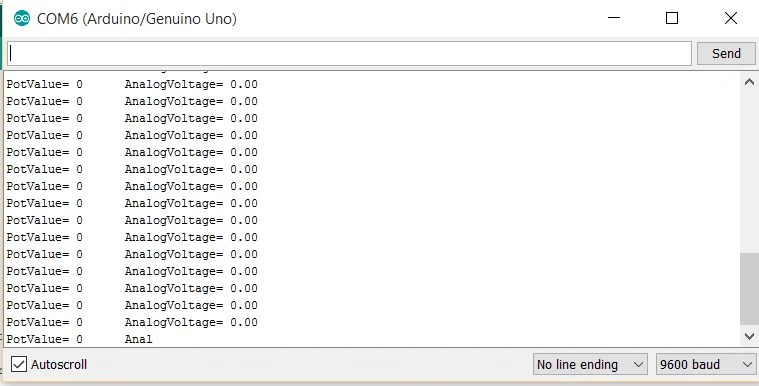3. If knob’s position is at 5 volt terminal then analog voltage will be 5 volts or analog value 1023 i.e, Minimum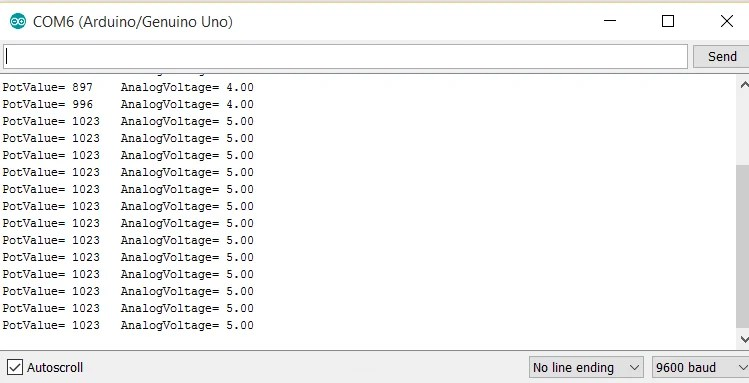Arduino Programming Basics: Program 3

Controlling Brightness of an LED using potentiometer:

In this project we are going to take analog input from one of the analog pins using a potentiometer and print these analog values as well as corressponding voltage value on Serial monitor :A) Connect end terminals of potentiometer to 5v and Gnd respectively whereas middle terminal to Analog pin A0.

B)  Connect LED in series with 220-ohm resistor from Pwm pin 9 to GND.

C)  Positive terminal of LED to PWM pin 9 through the resistor and negative terminal to GND pin.

NOTE: Since the brightness of LED is to be controlled through PWM, the LED must be connected to a PWM pin only.

Program:

 int x; //define variable x as an integer int y; //define variable y as an integer                                     void setup() {                         pinMode(A0,INPUT); // Set pin A0 as Input pin pinMode(9,OUTPUT); //Set digital pin 9 as output pin               Serial.begin(9600);}void loop() { x = analogRead(A0); // analog value from the potentiometer       y = map(x,0,1023,0,255); // converting the input analog value x(between 0 to 1023) into a PWM value between 0 to 255. This is called Mapping  analogWrite(9,y); // Giving this mapped value as PWM value to PWM pin 9. Remember that maximum PWM value is 255 i.e, 5 volts                  Serial.print(“PotValue= “); // giving a name to the value to be printed Serial.print(x); // printing potentiometer analog input       Serial.print(“\t”); // Next variable will be printed in next column                            Serial.print(” PWM Value= “); // giving a name to the value to be printed Serial.print(y); // printing PWM value that corresponds to an input analog value from the potentiometer        Serial.println(); // variable’s value will be printed on next line or row                            delay(500); // time difference bw successive print value }

**Explanation of the code is in comments itself.

Working:

1. Voltage across end terminals of the potentiometer is 5 volts and between the middle terminal i.e, analog output and Gnd terminal depends on knob’s position.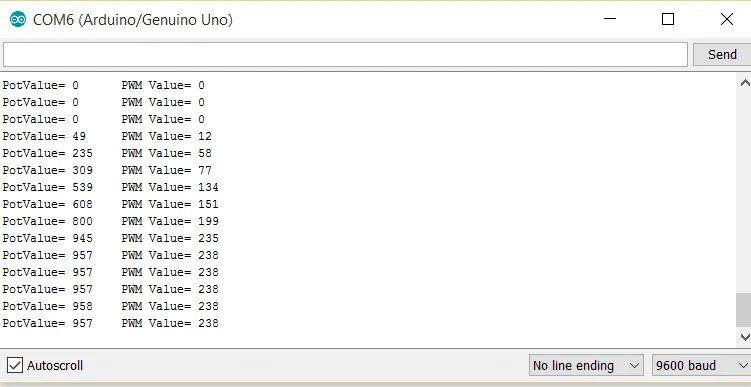2. If knob’s position is at Gnd terminal then analog voltage will be 0 or analog value 0 i.e, Minimum. This is equals to 0 PWM value(using Map function). This PWM value is given to pin 9 and hence brightness of LED is minimum.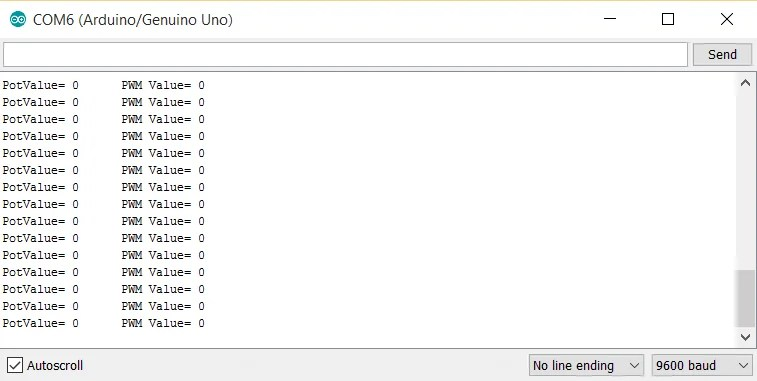3. If knob’s position is at 5 volt terminal then analog voltage will be 5 volts or analog value 1023 i.e, Minimum. This is equals to 255 PWM value(using Map function). This PWM value is given to pin 9 and hence brightness of LED is maximum.